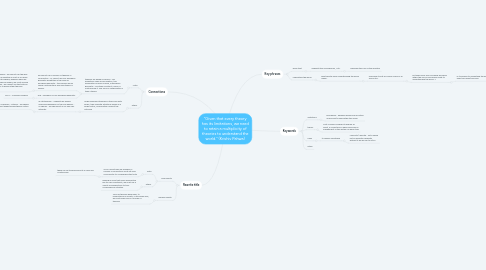# “Given that every theory has its limitations, we need to retain a multiplicity of theories to und...Krishiv PAHWA
Get Started. It's Free“Given that every theory has its limitations, we need to retain a multiplicity of theories to understand the world.” (Krishiv Pahwa)## 1. Key phrases

### 1.1. given that

1.1.1. suggests this is predefined , is it?

1.1.1.1. challenge the core of the question

### 1.2. understand the world

1.2.1. what exactly does understanding the world mean?

1.2.1.1. assuming its just an overall scope of all AOK's etc?

1.2.1.1.1. but does every AOK and each discipline within the AOK's necessarily relate to understanding the world...?

## 2. Keywords

### 2.1. limitations

2.1.1. boundaries - perhaps referencing a limited scope and/or gaps within the scope

### 2.2. theory

2.2.1. a set of ideas compiled to explain an event, a conjecture in which each idea is independent of the system of ideas itself

### 2.3. need

2.3.1. to require something

2.3.1.1. concrete / definite - not a desire but an absolute necessity, without it we will fail to do so.

## 3. Connections

### 3.1. Math

3.1.1. theories are based on axioms - we essentially make an assumption, this assumption is given a name, ie euclidean geometry - and then construct a form of math around it. This form of mathematics is then a theory

3.1.1.1. we cannot use a number of theories in conjunction - ie, cannot use non-euclidean geometry properties in the realm of euclidean geometry - this layover would cause contradictions and each theory is unique.

3.1.1.1.1. simple example - we cannot use the idea of rooting a negative in most of our basic fundamental algebra, however when we study complex numbers, we must assume that we can - we cannot use the truths in one theory to define other theories though.

3.1.1.2. RLE - euclidean vs non euclidean geometry

3.1.1.2.1. RLE 2 - Complex numbers

### 3.2. Ethics

3.2.1. Single ideas and theories in ethics are quite binary, they evaluate situations based on a single metric, ie absolutism looks at the outcome

3.2.1.1. ie utilitarianism - suggests we should maximise happiness for the max people - or egoism - we should act in our own self interests.

3.2.1.1.1. opposite of egoism - altruism - we should give without expecting anything in return

## 4. Rewrite title

### 4.1. AOK rewrite

4.1.1. math

4.1.1.1. As all conjectures are bounded, a number of conjectures must be used concurrently to comprehend the truth.

4.1.1.1.1. these can be transformed into a claim and counterclaim

4.1.2. Ethics

4.1.2.1. Bearing in mind that every perspective has its own constraints, we must use a variety of perspectives to truly comprehend a situation.

### 4.2. general rewrite

4.2.1. Since all theories have gaps, to understand our society, in any given area, we must make use of a myriad of theories